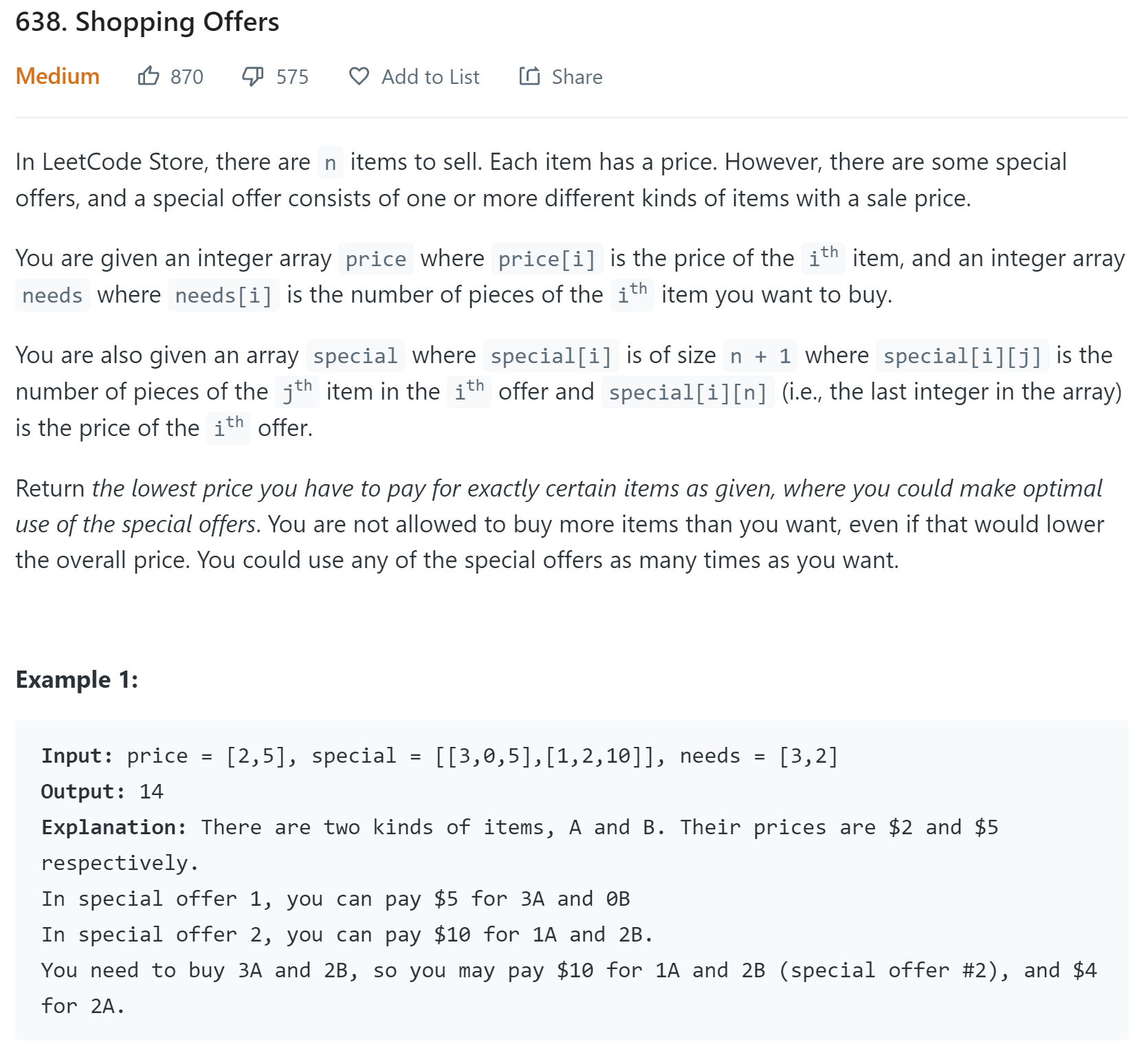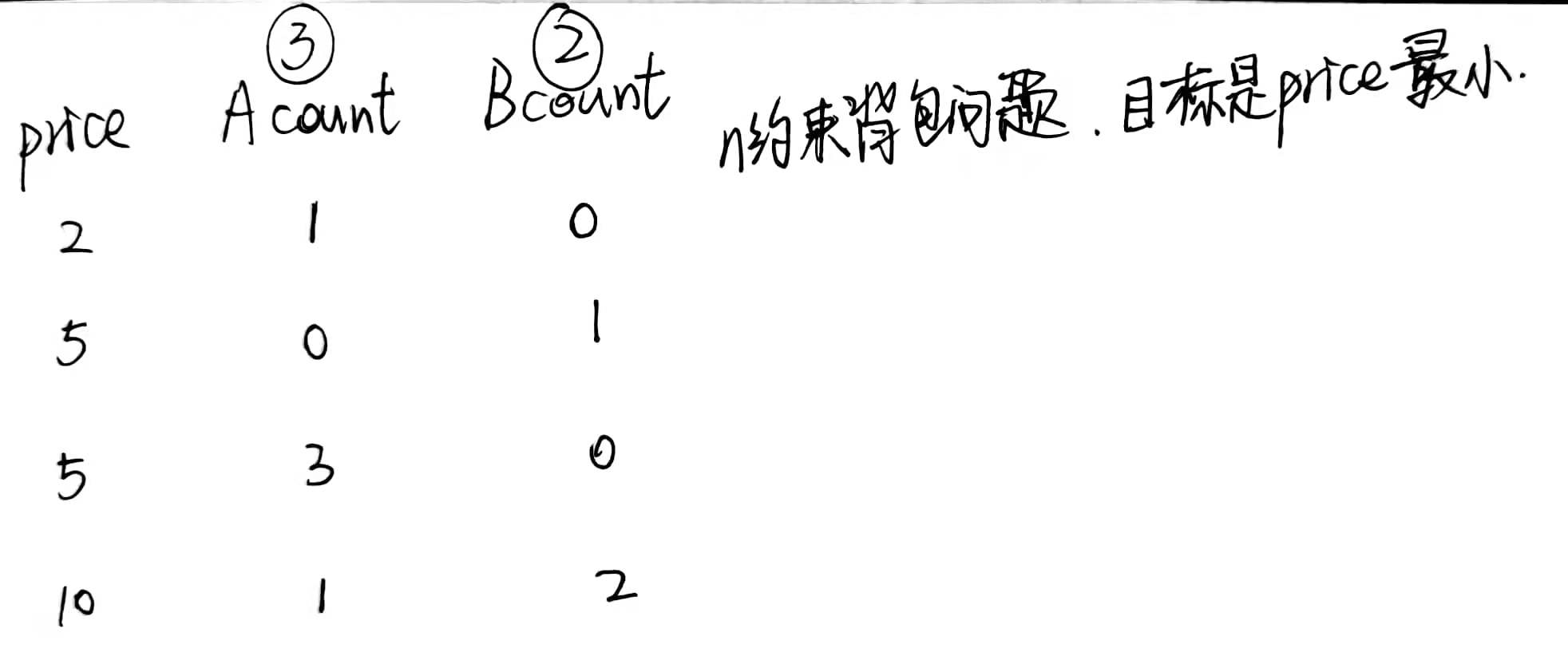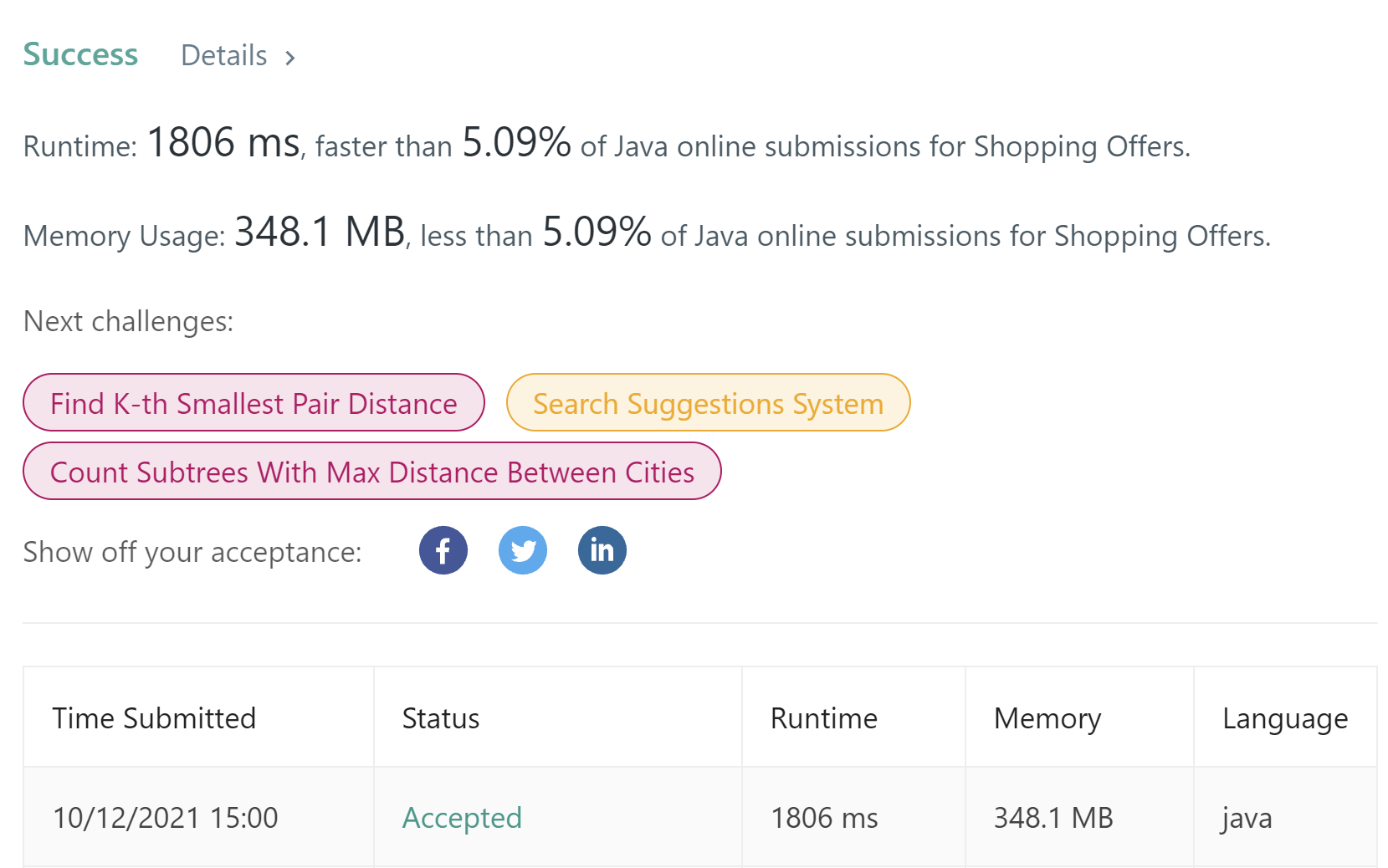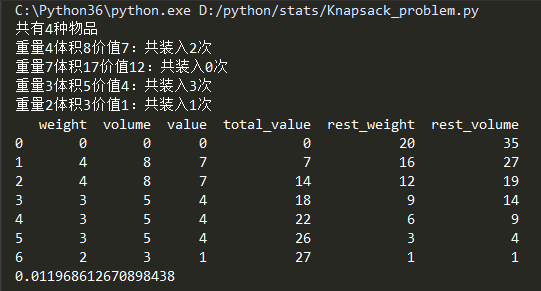• 采用混合遗传算法求解多约束背包问题．首先构建多约束背包问题的数学模型，然后采用多维实数编码方式的遗传算法，结合附带染色体库技术、局部启发式算子和扰动算子对问题进行求解，并给出了一个实验实例．实验证明...
• 实现二重约束背包问题c++代码： /**输入参数： * @param m 表示背包的最大容量 * @param m 表示背包的最大质量 * @param n 表示商品个数 * @param a[] 每个商品的容量 * @param b[] 每个商品的质量 * @...
• 一和零（双约束背包问题） 本题实质上是一个约束（n<6）的背包问题，求解目标是，在背包刚好装满的情况下，希望让总的 price 最小。物品可以使用无限次。 参考左神体系班学习班第19节：剪贴纸问题（实际上没...

题目

https://leetcode.com/problems/shopping-offers/题解

本题实质上是一个多约束（n<6）的背包问题，求解目标是，在背包刚好装满的情况下，希望让总的 price 最小。物品可以使用无限次。

参考左神体系班学习班第19节：剪贴纸问题（实际上没参考它，只是想到有这么个题）

给定一个字符串str，给定一个字符串类型的数组arr，出现的字符都是小写英文
arr每一个字符串，代表一张贴纸，你可以把单个字符剪开使用，目的是拼出str来
返回需要至少多少张贴纸可以完成这个任务。
例子：str= “babac”，arr = {“ba”,“c”,“abcd”}
ba + ba + c 3 abcd + abcd 2 abcd+ba 2
所以本题返回2

由于一些物品是以组合的形式给出的，所以我们先将 list 嵌套 list 转化成数组，方便索引查找。
然后，因为物品可以单独购买。为了统一方便，我们将可以单独购买的物品也转化成组合的形式，只不过让它们的系数都为 1。

如下图所示，前两行分别是单独购买 A 物品、单独购买 B 的价格，后两行是 AB 物品组合购买的价格。然后就是经典的 暴力递归 -> 傻缓存了。本题应该是无法转化成 dp 的，因为它的状态太多了，详见 dp map 中的 key，我的处理方式是将状态压缩成了字符串。后来看题解发现，hashmap 可以直接用 list 作为 key。

还有，我这个效率超级低，（应该是我为了避免回溯而copy的数组开销比较大），删掉 import 的头文件的话，会超时（这又是 leetcode 的蜜汁特性，带头文件的话运行速度快一些），带着头文件可以 AC。

import java.util.HashMap;
import java.util.List;
import java.util.Map;

class Solution {
int M; // 套餐总数
int N; // 物品总数

public int shoppingOffers(List<Integer> price, List<List<Integer>> special, List<Integer> needs) {
N = price.size();
M = special.size() + price.size();

// 数组化 套餐列表
int[][] sp = new int[M][N + 1];
for (int i = 0; i < special.size(); i++) {
List<Integer> list = special.get(i);
for (int j = 0; j < N + 1; j++) {
sp[i][j] = list.get(j);
}
}
for (int i = special.size(), j = 0; i < M; i++, j++) {
sp[i][j] = 1;
sp[i][N] = price.get(j);
}

// 数组化 购物清单
int[] need = new int[N];
for (int i = 0; i < N; i++) {
need[i] = needs.get(i);
}

Map<String, Integer> dp = new HashMap<>();
return process(sp, need, 0, dp);
}

// 多约束背包问题
// 物品列表在special中。当前可选择的物品在cur位置，还剩余needs要买的情况下，至少需要多少cost
public int process(int[][] special, int[] needs, int cur, Map<String, Integer> dp) {
// 查缓存
StringBuilder key = new StringBuilder();
for (int n : needs) { // 状态压缩成字符串
key.append(n).append(":");
}
key.append(cur);
if (dp.containsKey(key.toString())) return dp.get(key.toString());

if (allZero(needs)) {
dp.put(key.toString(), 0);
return 0;
}

int minCost = Integer.MAX_VALUE;
for (int p = cur; p < M; p++) { // 当前购买special[p]套餐
for (int count = 0; canBuy(special, needs, count, p); count++) { // 购买count件p套餐
int[] newNeeds = buy(special, needs, count, p);
int newCost = process(special, newNeeds, p + 1, dp);
if (newCost != Integer.MAX_VALUE) {
minCost = Math.min(minCost, count * special[p][N + 1 - 1] + newCost);
}
}
}
// 缓存
dp.put(key.toString(), minCost);

return minCost;
}

// 当前状态下，如果继续买count件p物品，是否买了不需要的
public boolean canBuy(int[][] special, int[] needs, int count, int p) {
for (int k = 0; k < N; k++) {
if (needs[k] - count * special[p][k] < 0) return false;
}
return true;
}

public int[] buy(int[][] special, int[] needs, int count, int p) {
int[] newNeeds = new int[N];
for (int k = 0; k < N; k++) {
newNeeds[k] = needs[k] - count * special[p][k];
}
return newNeeds;
}

public boolean allZero(int[] needs) {
for (int n : needs) {
if (n != 0) return false;
}
return true;
}

}展开全文leetcode 动态规划
• 基于线性规划解决二重约束完全背包问题，c++代码。实现功能： 输入： 1、背包容量V质量M和物品数量n 2、//每个物品的容量v[i]和质量m[i] 输出： 最大value
• 多重背包问题

2020-12-31 03:53:54
悼念512汶川大地震遇难同胞——珍惜现在，感恩生活 Time Limit: 1000/1000 MS (Java/Others) Memory Limit: 32768/32768 K (Java/Others) Total Submission(s)... } 因为是多背包，可能在第j种的时候已经选了s个，并且s

悼念512汶川大地震遇难同胞——珍惜现在，感恩生活

Time Limit: 1000/1000 MS (Java/Others) Memory Limit: 32768/32768 K (Java/Others)

Total Submission(s): 12417 Accepted Submission(s): 5250

Problem Description

急！灾区的食物依然短缺！

为了挽救灾区同胞的生命，心系灾区同胞的你准备自己采购一些粮食支援灾区，现在假设你一共有资金n元，而市场有m种大米，每种大米都是袋装产品，其价格不等，并且只能整袋购买。

请问：你用有限的资金最多能采购多少公斤粮食呢？

后记：

人生是一个充满了变数的生命过程，天灾、人祸、病痛是我们生命历程中不可预知的威胁。

月有阴晴圆缺，人有旦夕祸福，未来对于我们而言是一个未知数。那么，我们要做的就应该是珍惜现在，感恩生活——

感谢父母，他们给予我们生命，抚养我们成人；

感谢老师，他们授给我们知识，教我们做人

感谢朋友，他们让我们感受到世界的温暖；

感谢对手，他们令我们不断进取、努力。

同样，我们也要感谢痛苦与艰辛带给我们的财富～

Input

输入数据首先包含一个正整数C，表示有C组测试用例，每组测试用例的第一行是两个整数n和m(1<=n<=100, 1<=m<=100),分别表示经费的金额和大米的种类，然后是m行数据，每行包含3个数p，h和c(1<=p<=20,1<=h<=200,1<=c<=20)，分别表示每袋的价格、每袋的重量以及对应种类大米的袋数。

Output

对于每组测试数据，请输出能够购买大米的最多重量，你可以假设经费买不光所有的大米，并且经费你可以不用完。每个实例的输出占一行。

Sample Input

1

8 2

2 100 4

4 100 2

Sample Output

400

#include#include

#define MAXN 110

#define MAXM 110

using namespacestd;struct GOODS{ intprice, weight, quantity; };

GOODS goods[MAXN];//行代表第i种，列代表还有j元钱

int n, m;//种类，钱

intdp[MAXN][MAXM];intmain()

{intt;

cin>>t;while (t--){

cin>> m >>n;for (int i = 1; i <= n; i++){

cin>> goods[i].price >> goods[i].weight >>goods[i].quantity;

}

memset(dp,0, sizeof(dp));for (int i = 1; i <= n; i++){for (int j = 0; j <= m; j++){for (int k = 0; k <= goods[i].quantity&&k*goods[i].price <= j; k++){

dp[i][j]= max(dp[i][j], dp[i-1][j - k*goods[i].price] + k*goods[i].weight);//好好想想为什么这里不是dp[i-1][j]

}

}

}

cout<< dp[n][m] <

}return 0;

}

因为是多背包，可能在第j种的时候已经选了s个，并且s

展开全文多维多重背包问题
• dp——多约束背包 一、题目描述 题目 N件物品和一个容量是 V的背包，背包能承受的最大重量是 M。每件物品只能用一次。体积是 vi，重量是 mi，价值是 wi。求解将哪些物品装入背包，可使物品总体积不超过背包容量，总...

dp——多约束背包

一、题目描述

题目

N件物品和一个容量是 V的背包，背包能承受的最大重量是 M。每件物品只能用一次。体积是 vi，重量是 mi，价值是 wi。求解将哪些物品装入背包，可使物品总体积不超过背包容量，总重量不超过背包可承受的最大重量，且价值总和最大。输出最大价值。

输入格式

第一行三个整数，N，V,M，用空格隔开，分别表示物品件数、背包容积和背包可承受的最大重量。接下来有 N 行，每行三个整数 vi,mi,wi，用空格隔开，分别表示第 i 件物品的体积、重量和价值。

输出格式

输出一个整数，表示最大价值。

4 5 6
1 2 3
2 4 4
3 4 5
4 5 6

8

数据范围

0<N≤100，0<V<1000, 0<M≤1000, vi,mi,wi都小于10000

二、解题思路

因为题目是最优子结构问题，所以可以采用动态规划来做。

【数学建模】

令dp[i][j][k]表示：前i个物品，当背包体积为j，背包能承受的重量为k，时的可以获得的最多的价值

【状态方程】

因为这种背包每种物品只能拿一个，所以可以看成一种类似于01背包的背包问题。所以状态方程也可以参考01背包的来写：
dp[i][j][k]=max(dp[i-1][j][k],dp[i-1][j-V[i]][k-m[i]]+value[i]);

注意

如果j<V[i]或者k<m[j]那么状态转移方程就是：dp[i][j][k]=dp[i-1][j][k]

原因

这个状态转移方程得到的原因如下：

从第i个考虑，比较选还是不选。如果选择第i个物品，那么背包的体积就要≥这个物体的体积，且背包的重量≥物体的重量，最后再加上这个物体的价值，即：dp[i-1][j-V[i]][k-m[i]]+value[i]；如果此背包不满足：背包的体积≥这个物体的体积，且背包的重量≥物体的重量，则放弃这一个物品，即：dp[i-1][j][k]。

【边界（初始化）】

如果背包的重量或者背包的体积或者选择的物品数量为0，那么背包所能获得的最大价值就是0。所以背包的边界就是dp[i][j]=dp[i][k]=dp[j][k]=0

三、时间复杂度及扩展

时间复杂度

因为需要使用三重for循环，则可以粗略计算时间复杂度就是O(nVm)

扩展

如果这个背包的约束不仅仅只有两个而有多个的话，那么时间复杂度也会增长，空间复杂度也会增加，并且没有优化策略

四、代码

#include<iostream>
#include<cstdio>
using namespace std;
int n,V,m,dp;
struct node{
int V,m,val;
}A;
void init()
{
scanf("%d%d%d",&n,&V,&m);
for(int i=1;i<=n;i++)
scanf("%d%d%d",&A[i].V,&A[i].m,&A[i].val);
}
void solve()
{
for(int i=0;i<=n;i++)//初始化
{
for(int j=0;j<=n;j++)
{
for(int k=0;k<=n;k++)
if(i==0||j==0||k==0)
dp[i][j][k]=0;
}
}
for(int i=1;i<=n;i++)
{
for(int j=1;j<A[i].V;j++)//背包的体积不够
for(int k=1;k<=m;k++)
dp[i][j][k]=dp[i-1][j][k];
for(int j=A[i].V;j<=V;j++)
{
for(int k=1;k<A[i].m;k++)//背包所能承受的重量不够
dp[i][j][k]=dp[i-1][j][k];
for(int k=A[i].m;k<=m;k++)
dp[i][j][k]=max(dp[i-1][j-A[i].V][k-A[i].m]+A[i].val,dp[i-1][j][k]);
}
}
printf("%d",dp[n][V][m]);
}
int main()
{
init();
solve();
return 0;
}

展开全文动态规划 c++
• 问题描述：1、一个背包，往里装东西，物品重量w(weight)对应为[2,3,4,7] ，价值va(value)对应为[1,4,7,12] ，如果你的最大承重为20，每个物品可装次数不限，求你能装入背包的最大价值。 2、如果物品可装次数times...

问题描述：1、一个背包，往里装东西，物品重量w(weight)对应为[2,3,4,7] ，价值va(value)对应为[1,4,7,12] ，如果你的最大承重为20，每个物品可装次数不限，求你能装入背包的最大价值。
2、如果物品可装次数times对应为[4,3,2,1]，求你能装入背包的最大价值。
3、如果物品体积vo(volume)对应为[3,5,8,17]，最大容量为35，求你能装入背包的最大价值。

import pandas as pd

def Knapsack(w, vo, va, times, total_weight, total_volume):

total_value = 0
result = [{
'weight': 0,
'volume': 0,
'value': 0,
'total_value': total_value,  #递增
'rest_weight': total_weight,  #递减
'rest_volume':total_volume  #递减
}]

y =  * len(w)  #用以统计物品装入次数
for i in range(1000):  #设置尝试装入次数
k = 0
for j in range(len(w)):
if w[k] > total_weight or vo[k] > total_volume or y[k] == times[k]:  #去掉重量超过剩余承重或体积大于剩余容量或装入次数达到上限的物品
print("重量%d体积%d：共装入%d次" %(w[k], vo[k], y[k]))
del w[k], vo[k], va[k], times[k], y[k]
else:
k += 1

if len(w) > 0:
u = [[], []]
for j in range(len(w)):
u.append(min(times[j] - y[j], (total_weight // w[j]), (total_volume // vo[j])))
u.append(u[j] * va[j])  #第一次取整计算物品的最高可装入价值（第一层）

s = [[], []]
for k in range(len(w)):
if k == j:
s.append(0)
else:
s.append(min(times[k] - y[k], (total_weight - (w[j] * u[j]))// w[k], (total_volume - (vo[j] * u[j])) // vo[k]))
s.append(s[k] * va[k])  #对取整后的剩余重量、体积、次数再次取整计算物品的最高可装入价值（第二层）

t = [[], []]
for l in range(len(w)):
if l == j or l == k:
t.append(0)
else:
t.append(min(times[l] - y[l], (total_weight - (w[j] * u[j]) - (w[k] * s[k])) // w[l],(total_volume - (vo[j] * u[j]) - (vo[k] * s[k])) // vo[l]))
t.append(t[l] * va[l])  # 对取整后的剩余重量、体积、次数再次取整计算物品的最高可装入价值（第三层），层数越多，计算越准确，暂时只计算三层
s[k] += max(t)  #将第三次取整计算物品的最高可装入价值的最大值加到第二次的结果中
u[j] += max(s)  #将第二次取整计算物品的最高可装入价值的最大值加到第一次的结果中

if max(u) > 0:   #选择评估价值最高的物品优先装入
y[u.index(max(u))] += 1  #记录物品装入次数
weight, volume, value = w[u.index(max(u))], vo[u.index(max(u))], va[u.index(max(u))]
total_weight = total_weight - weight  #计算剩余承重
total_volume = total_volume - volume  #计算剩余容量
total_value += value  #计算已装入物品总价值
result.append({
'weight': weight,
'volume': volume,
'value': value,
'total_value': total_value,
'rest_weight': total_weight,
'rest_volume':total_volume
})
else:  #一旦无满足约束的物品可装入，则停止装入
break

return pd.DataFrame(result,columns = ['weight', 'volume', 'value', 'total_value', 'rest_weight', 'rest_volume'])

data['unitw_value'] = data['value'] / data['weight']
data = data.sort_values(by = 'unitw_value', ascending = False)

weight = data['weight'].tolist()
volume = data['volume'].tolist()
value = data['value'].tolist()
times = data['times'].tolist()
total_weight = 20
total_volume = 35

if __name__ == "__main__":
start_time = time.time()
print("共有%d种物品" %(len(weight)))
print(Knapsack(weight, volume, value, times, total_weight, total_volume))
end_time = time.time()
print(end_time - start_time)展开全文• 背包问题的模拟退火算法

千次阅读 2019-01-21 10:46:11
下面来介绍一下非常经典的背包问题及其用模拟退火算法实现的程序： 基本的背包向题,更复杂一些的比如:物品允许部分带走或者每类物品有个等情况，在这个背包的例子中,假设有12件物品,质量分别为2磅、4磅、11磅、13...背包问题 模拟退火算法
• 问题：假设有6个背包，有10个属性，要求出最优解，先已给出输出的价值，需要设计一个算法。 代码： import numpy as np #引用numpy包，存储和处理矩阵 from pulp import LpProblem, LpVariable, LpConstraint,...多维背包问题 遗传算法 python 算法 pycharm
• 文章目录 题目描述 问题求解 1.1 动态过程 1.2 代码求解 算法优化 1.1 空间优化 1.2 算法加速 算法约束 恰好放满背包 完整代码测试 写在前面 这是我对动态规划一些入门题的笔记，主要便于随时随地的回顾这些基础内容...动态规划 背包问题
• 以前写背包问题是没有考虑过装东西的先后顺序的，这题由于那个奇怪的商人，要装东西要有先后顺序。 我们假设买a b两样东西设其属性为 c1（cost）q1 v1 c2 q2 v2 先买a的话我们需要 c1 + q2 的钱 先买b的话我们...acm竞赛
• POJ 3132 双约束背包问题

千次阅读 2008-10-26 17:44:00
多重背包的问题是，给定物品，且有物品的数量...或者说，这里的背包有两重的约束，即背包的容量以及背包最多能装的物品的个数都有限制，不妨成为“双约束背包”。 这种背包问题的思考方式是这样的：用两维背包，pack
• 01背包问题 1.问题重述 给定n个物品，价值分别是：v1,v2,…,vn,重量分别是：w1,w2,…,wn。在物品不可分割的情况下，挑选物品放入承重为W的背包，使得背包内物品的价值最大，且背包内物品的总重量小于W. 2.解决方案 ...
• 背包问题不单单是一个简单的算法问题，它本质上代表了一大类问题，这类问题实际上是01线性规划问题，其约束条件和目标函数如下： 自从dd_engi在2007年推出《背包问题九讲》之后，背包问题的主要精髓基本已道尽。...
• 这是我自己实现的，包括贪心算法和动态规划等解决方法，真的很实用背包问题
• 遗传算法的有趣应用很，诸如寻路问题，8数码问题，囚犯困境，动作控制，找圆心问题（在一个不规则的多边形中，寻找一个包含在该多边形内的最大圆圈的圆心），TSP问题，生产调度问题，人工生命模拟等。下面我以...
• 该装备实现：? - 在一定约束背包总容量）下，从数百种物品中选取出最优组合，使得总价值最大? -输出背包所能承载的物品组合的最大总价值，并给出所选物品的序号、价值和重量
• 将部分穷举法与贪婪算法相结合，给出求解多背包约束下非减下模集函数最大值的近似算法。证明了该算法的性能保证是1－e－1，算法的时间复杂性为O（3n4）。
• 提出一种处理高维背包问题(KP) 的贪婪封装二进制差分进化算法(GPBDE), 并设计了一种贪婪封装的修补策略处理不可行解. 为了提高种群的多样性及算法的全局搜索能力, 对适应度较低的个体执行对偶变换. 数值实验选取4 种...
• 如何使用MKP启发式求解器 ... 仅当您希望在输出消息中隐藏目标，约束，所选项目和废弃项目时，请注意这一点。 让我们明确说明如何调用十二种算法中的每一种： $# Genetic algorithm$ mkp < path>
• 01背包问题（变形） 有一个箱子容量为v(正整数,o≤v≤20000),同时有n个物品(o≤n≤30),每个物品有一个体积 (正整数).要求从 n 个物品中,任取若干个装入箱内,使箱子的剩余空间为最小. 输入 第一行,一个整数,表示...c++ 算法
• 贪心粒子群算法求解多维0-1背包问题，郝俊玲，，本文将单维背包问题求解中常用的贪心思想推广到多维0-1背包问题，但多维背包问题多约束特性使得单维背包问题中按物品性价比非增多维背包问题
• 多维多选的背包问题

万次阅读 2017-11-12 15:47:18
0-1背包问题是一类典型的组合优化问题，它要求找出n个物体的一个子集使其尽可能的装满容量为W的背包...多约束背包问题等。选择背包问题定义为有附加约束条件的背包问题，该问题带有互不相关的选择约束。该问题的特背包问题
• python背包问题

2020-11-23 23:40:47
广告关闭腾讯云11.11云上盛惠 ，精选热门产品助力上云，云服务器首年88元起，买的越返的越，最高返5000元！动态规划会强调“状态”，通过自定义的一维或二维数组为我们将物品装入背包这个行为定义成状态的变化，...
• 0/1多维背包问题（0/1 MKP）是一个有趣的NP-hard组合优化问题，可以对物流，金融，电信和其他领域中许多具有挑战性的应用程序进行建模。 在0/1 MKP中，给出了一组项目，每个项目都有大小和值，必须将其放入具有一定...
• 双重背包问题

千次阅读 2019-06-27 23:39:15
i++) { //条件编译，表示背包可以不存储满 for(j=1;j;j++) { result[i][j] = INF ; result = 0 ; } } for(k=0;k;k++) { for(i=reactorCap;i >= volumes[k];i--) { //i对应reactorCap ...
• 有时会遇到这样一类题目，它的问题可以分解，但是又不能得出明确的动态规划或是递归解法，此时可以考虑用回溯法解决此类问题。回溯法的优点 再于其程序结构明确，可读性强，易于理解，而且通过对问题的分析...
• 什么是属性的背包问题？比如对于这道题，它可以看作是一个具有装下物品两个属性的背包(装下0和1)，它的容量可以看作是[j][k]可用二维数组来表示，而外面一层和普通的01背包问题一样，用于表示在有多少个物体的情况...动态规划 字符串 数据结构
• 针对多维背包问题(MKP) NP-hard、约束强的特点, 提出一种高效的蚁群-拉格朗日松弛(LR) 混合优化算法. 该算法以蚁群优化(ACO) 为基本框架, 并基于LR 对偶信息定义了一种MKP效用指标. ACO使得整体算法具有全局搜索能力......